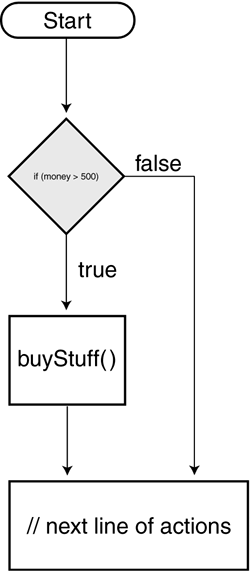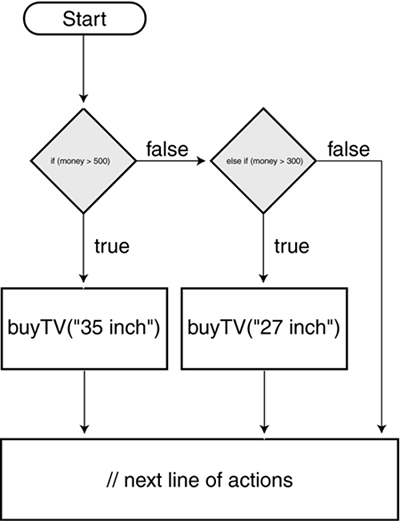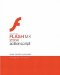# Controlling a Script s Flow

### Controlling a Script's Flow

Typically, actions in your scripts execute consecutively, from beginning to end a sequence that represents your script's flow. Using conditional logic, you can control this flow by scripting specific actions to execute only when specific conditions are met or exist in your movie. By implementing conditional logic in your scripts, you give your movie the ability to make decisions and take action based on various conditions you've set, and your movie takes on more dimension as a result. You'll use the conditional statements or phrases described in this lesson to implement conditional logic in your scripts.

#### If/Then Statements

At the heart of conditional logic is the simple if/then statement. Here's an example:

` if (moneySaved > 500) { buyStuff(); } // next line of actions... `

The buyStuff() function is called only if the variable moneySaved has a value greater than 500. If moneySaved is equal to or less than 500, the buyStuff() function call is ignored and actions immediately below the if statement are executed.At its core, a conditional statement looks at a circumstance (placed within parentheses) and determines whether that circumstance is true or false. If the circumstance is true, actions within the statement are executed; if the circumstance is false, the actions are ignored. When you create an if statement, you state, essentially:

` if (...what is shown here is true) {   Do this; } `

The data you place within parentheses represents the condition to be analyzed. The data within the curly braces ({}) represents the actions to be taken if the condition exists.

As in real life, sometimes an if statement needs to analyze multiple conditions before taking a single action or set of actions. For example:

` if (moneySaved > 500 && billsPaid == true) {   buyStuff(); } `

The AND operator (&&) has been added to the statement so that now the buyStuff() function is called only if moneySaved is more than 500 and billsPaid has a value of true. If either condition is false, buyStuff() will not be called.

Using the OR operator (||) allows you to take a slightly different approach:

` if (moneySaved > 500 || wonLottery == true) {   buyStuff(); } `

The buyStuff() function is called if either moneySaved has a value greater than 500 or wonLottery has a value of true. Both conditions need not be true for the buyStuff() function to be called, as was the case when using the AND operator (&&) in the earlier example.

You can mix the AND and OR operators to create sophisticated conditional statements like this one:

` if (moneySaved > 500 && billsPaid == true || wonLottery == true) {   buyStuff(); } `

In this script, the buyStuff() function is called only if moneySaved is more than 500 and billsPaid has a value of true, or if wonLottery has a value of true.

The following table shows a list of the common operators (known as comparison operators because they're used to compare values) used in conditional logic, with brief descriptions and examples of how they're used.

OPERATOR

DESCRIPTION

EXAMPLE

EXECUTE THE FUNCTION IF…

==

Checks for equality

if (name == "Derek")

name has an exact value of Derek

!=

Checks for inequality

if (name != "Derek")

name has a value other than Derek

<

Less than

if (age < 30)

age has a value less than 30

>

Greater than

if (age > 30)

age has a value greater than 30

<=

Less than or equal to

if (age <= 30)

age has a value less than or equal to 30

>=

Greater than or equal to

if (age >= 30)

age has a value greater than or equal to 30

&&

Logical AND

if (day == "Friday" && pmTime > 5)

day has a value of Friday and pmTime has a value greater than 5

||

Logical OR

if (day == "Saturday" || day == "Sunday")

day has a value of Saturday or Sunday

A common mistake when checking equality is to insert a single equals sign (=) where a double equals sign (==) belongs. Use a single equals sign to assign a value (for example, money = 300). Use a double equals sign to check for equality: money == 300 does not assign a value of 300 to money. Rather, it asks whether money has a value of 300.

NOTE

Although number comparisons are straightforward after all, most of us understand that 50 is less than 100 text-value comparisons are less obvious. Derek doesn't equal derek even though the same letters are used. With string values, A has a lower value than Z, and lowercase letters have greater values than uppercase letters. Thus, if A has a value of 1, z has a value of 52 (ABCDEFGHIJKLMNOPQRSTUVWXYZabcdefghijklmnopqrstuvwxyz).

#### If/Else If Statements

An if/else if statement is similar to the basic if statement except that it enables your script to react to multiple conditions. Here's an example:

` if (money > 500) {   buyTV("35 inch"); } else if (money > 300) {   buyTV("27 inch"); } `This script executes various actions depending on the value of money: "If money has a value greater than 500, buy the 35-inch TV." If money has a value less than 500, this part of the script is ignored and the next condition is examined. The next condition says that if money has a value greater than 300, buy the 27-inch TV. Thus, if money has a value of 450 when this script is executed, the first part of the statement is ignored (because 450 is not greater than 500), but the second part of the statement is executed (because a value of 450 is greater than 300). If money has a value less than 300, both parts of this statement are ignored.

You can create several lines of if/else if statements that react to dozens of conditions.

#### If/Else Statements

An if/else statement allows your script to take action if no conditions in the statement prove true. Consider this the fail-safe part of the statement.

` if (money > 500) {   buyTV("35 inch"); } else if (money > 300) {   buyTV("27 inch"); } else {   workOvertime (); } `In this script, if money doesn't have a value of at least 300, neither of the conditions analyzed is true; as a result, neither of the actions that follow the conditions will be executed. Instead, the actions following the else part of the statement are executed it's a bit like saying this:

` if (...this is true) {   // Do this } otherwise, if (...this is true) {   // Do this } otherwise, if none of the conditions above is true {   // Do this } `

#### Conditional Statement Variations

The statements we've discussed so far (and the syntax used) form the basis of most of the conditional logic you'll use in your projects. However, as with most things in ActionScript, there's more than one way to script a conditional statement. Let's look at a couple of these variations and how you might use them to shorten your code and make it easier to read. The following variations have no programmatic advantage over the versions we've already discussed. How you take advantage of them is determined by personal preference.

A switch statement, which is a variation on the if/else statement, can be useful if you want a script to take a specific set of actions when an exact value exists. For example:

` switch (favoriteBand) { case "Beatles":   gotoAndPlay("Beatles");   break; case "U2":   gotoAndPlay("U2");   break; default:   gotoAndPlay("Slim Whitman"); } `

A single expression in parentheses (usually a variable) is evaluated once. The value of the expression is compared with the values for each case in the structure. If a match exists, the block of code associated with that case is executed. If no match is found, the default statement at the end is executed. break is used in each case to prevent the code from automatically running into the next case if a match has already been found. Each case can check string values (as shown in the example), numeric values, and Boolean values of true and false. (For Boolean values, however, the variation we describe next represents an easier and more appropriate technique.)

The ternary operator (?:) lets you write a simple if/else statement in one line. Here's an example:

` var myMood:String = ((money > 1000000) ? "Happy" : "Sad"); `

If the condition within the parentheses evaluates to true, the first expression ("Happy") is set as the value of myMood. If the condition evaluates to false, the second expression ("Sad") is used. This single line of ActionScript accomplishes the same thing as the following code:

` if (money > 1000000) {   myMood = "Happy"; } else {   myMood = "Sad"; } `

Here's an example of an extended way to use the ternary operator:

` var myMood:String  = "I'm " + ((money > 1000000) ? "happy" : "sad"); `

In this example, myMood will have a value of either "I'm happy" or "I'm sad" depending on whether the value of money is greater than or less than 1000000.

The ternary operator is commonly used to toggle variable states. Here's an example:

` var playSound:Boolean = (playSound) ? false : true; `

Every time this script is executed, the variable playSound is changed from true to false or from false to true.Macromedia Flash MX 2004 ActionScript: Training from the Source
ISBN: 0321213432
EAN: 2147483647
Year: 2005
Pages: 182

Similar book on Amazon

flylib.com © 2008-2017.
If you may any questions please contact us: flylib@qtcs.net